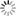# Episode List

OR

### 1983

26 Sep. 1983
The MonumentBe the first one to add a plot.

3 Oct. 1983
The Last DanceBe the first one to add a plot.

10 Oct. 1983
BanjoBe the first one to add a plot.

17 Oct. 1983
The TrialBe the first one to add a plot.

24 Oct. 1983
Second FiddleBe the first one to add a plot.

31 Oct. 1983
The DecisionBe the first one to add a plot.

14 Nov. 1983Be the first one to add a plot.

21 Nov. 1983Be the first one to add a plot.

5 Dec. 1983
The AuctionBe the first one to add a plot.

12 Dec. 1983
Words and Music